Question

4. Each morning a hospital quality-control person checks two pulse oximeters by mounting them on a testing rig that simulates a stable patient. Experience shows that the oximeters give normally distributed readings with variances that match closely and change little, while the mean readings drift from one another over time. In one morning’s check, simple random samples of 5 readings were taken from the first oximeter and of 7 readings from the second. The average oxygen level from the first oximeter was 98.3 with standard deviation 0.31, while the average from the second was 96.1 with standard deviation 0.29.

• Find a 98% confidence interval for the difference in population mean readings from the two oximeters.

• Test whether the population mean readings from the two oximeters are the same.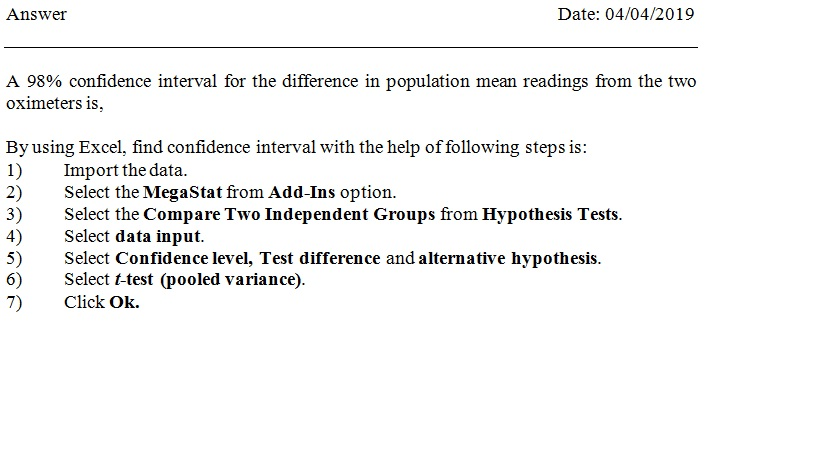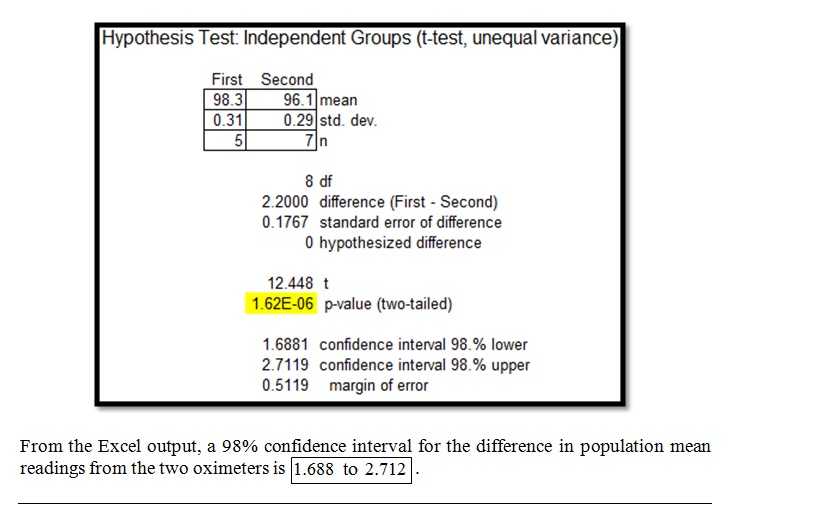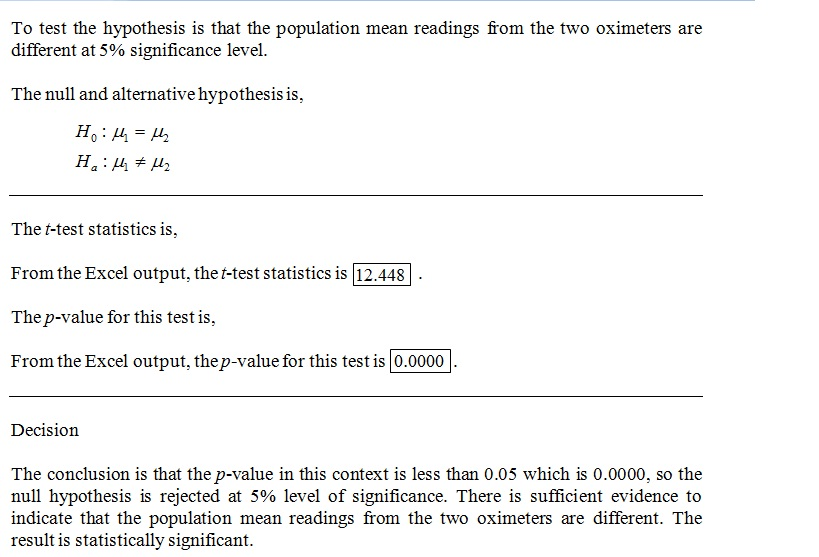#### Earn Coins

Coins can be redeemed for fabulous gifts.

Similar Homework Help Questions
• ### Two samples each of size 20 are taken from independent populations assumed to be normally distributed...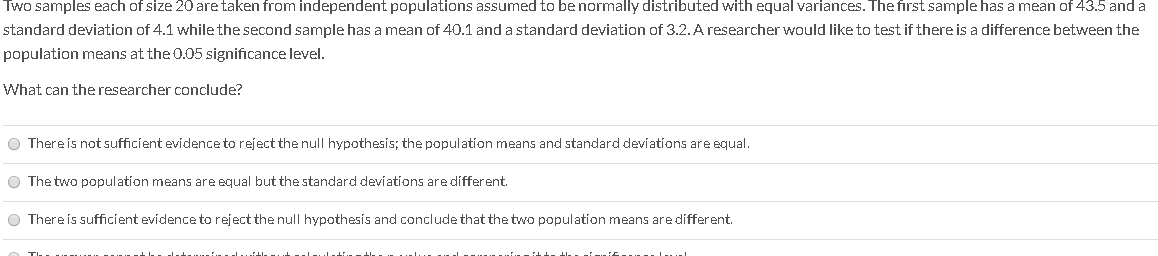Two samples each of size 20 are taken from independent populations assumed to be normally distributed with equal variances. The first sample has a mean of 43.5 and a standard deviation of 4.1 while the second sample has a mean of 40.1 and a standard deviation of 3.2. A researcher would like to test if there is a difference between the population means at the 0.05 significance level. What can the researcher conclude? There is not sufficient evidence to reject...

• ### photos for each question are all in a row (1 point) In the following questions, use...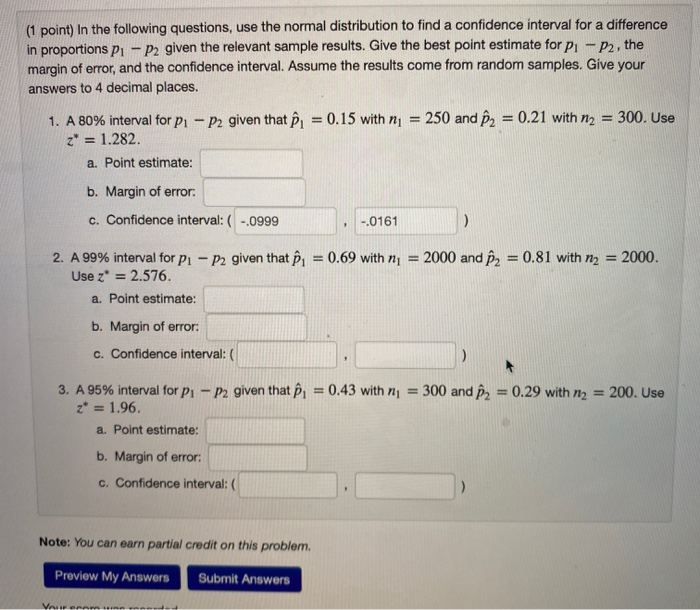photos for each question are all in a row (1 point) In the following questions, use the normal distribution to find a confidence interval for a difference in proportions pu - P2 given the relevant sample results. Give the best point estimate for p. - P2, the margin of error, and the confidence interval. Assume the results come from random samples. Give your answers to 4 decimal places. 300. Use 1. A 80% interval for pı - P2 given that...

• ### summatize the following info and break them into differeng key points. write them in yojr own...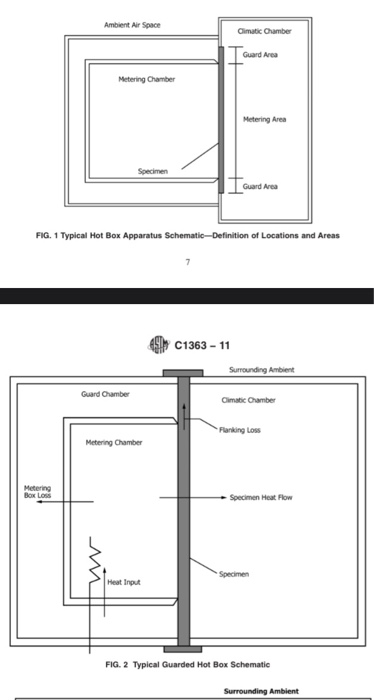summatize the following info and break them into differeng key points. write them in yojr own words   apartus 6.1 Introduction—The design of a successful hot box appa- ratus is influenced by many factors. Before beginning the design of an apparatus meeting this standard, the designer shall review the discussion on the limitations and accuracy, Section 13, discussions of the energy flows in a hot box, Annex A2, the metering box wall loss flow, Annex A3, and flanking loss, Annex...